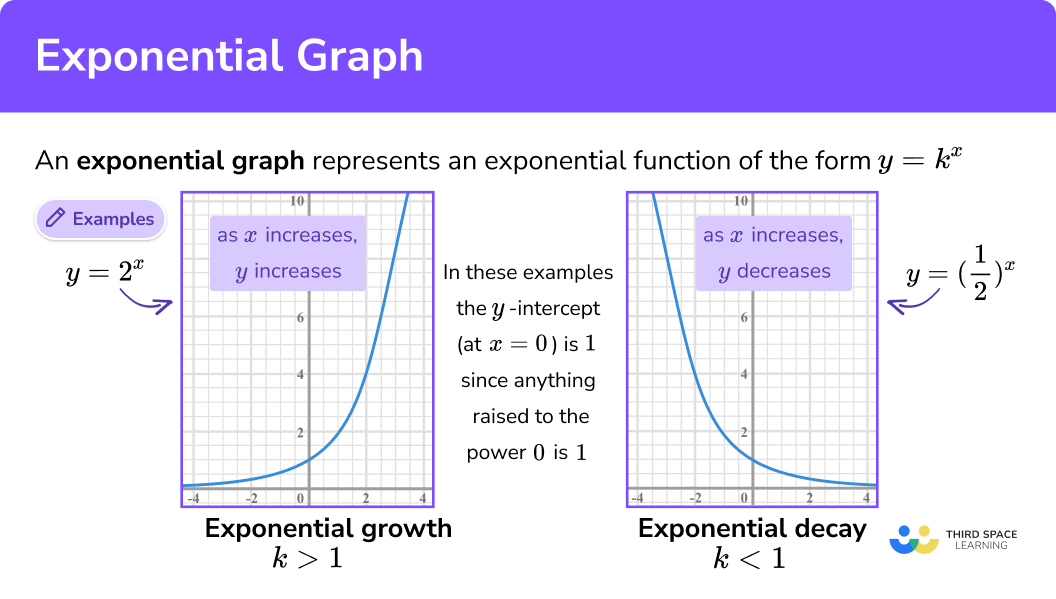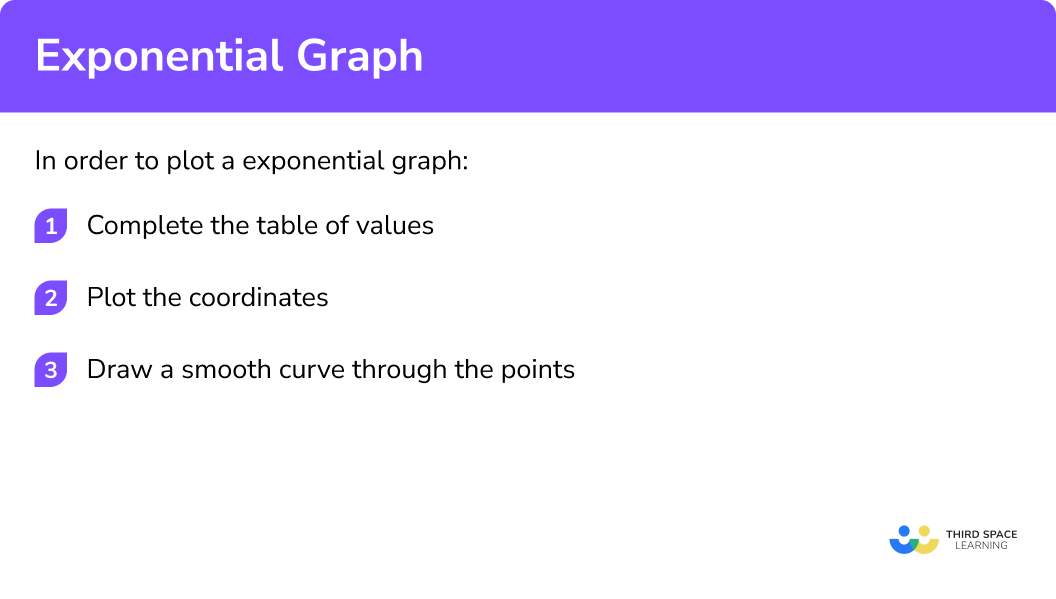GCSE Maths Algebra Types Of Graphs

Exponential Graph

# Exponential Graph

Here we will learn about exponential graphs, including recognising, sketching, plotting and interpreting exponential graphs.

There are also exponential graphs worksheets based on Edexcel, AQA and OCR exam questions, along with further guidance on where to go next if you’re still stuck.

## What is an exponential graph?

An exponential graph is a representation of an exponential function of the form

$y=k^x$

Where x and y are variables and k is a constant (a numerical value).
x is the exponent and k is the base.

The y-intercept of an exponential curve (at x = 0 ) is 1 since any non-zero number raised to the power 0 is 1.

The x-axis is an asymptote to the curve.
The curve gets very close to the horizontal asymptote but does not touch it. This is because y ≠ 0.

The graph of an exponential function can represent either exponential growth or exponential decay:

• When k is greater than 1 it is a growth curve. As x increases, so does y.
It can be used to represent population growth or compound interest.

E.g.

$y=2^x$

• When k is less than 1 it is a decay curve. As x increases, y decreases.
It can be used to represent radioactive decay.

E.g.

$y=(\frac{1}{2})^x$

There are more complex exponential functions of the form:

$y=ab^x$

The graphs look similar to the ones above, they have an exponent x, base b and the y-intercept is a.

E.g.

$y=4\times 3^x$

### What is an exponential graph?## Growth and decay problems

Exponential graphs can represent two types of problems, growth and decay. They involve real-life situations.

Growth problems are when the amount increases over time. An example is how a population increases over time.

Decay problems are when the amount decreases over time. An example is  the amount of radiation from a radioactive substance over time.

## How to recognise an exponential graph

In order to recognise an exponential graph:

1. Identify linear or quadratic or any other functions
2. Identify the exponential function

## Related lessons on types of graphs

Exponential graph is part of our series of lessons to support revision on types of graphs. You may find it helpful to start with the main types of graphs lesson for a summary of what to expect, or use the step by step guides below for further detail on individual topics. Other lessons in this series include:

## Recognising an exponential graph examples

### Example 1: recognise exponential graphs

Which is the correct equation for the graph?

$y=x^2+3 \quad \quad \quad y=3x+1 \quad \quad \quad y=\frac{3}{x} \quad \quad \quad y=3^x$

1. Identify linear or quadratic or any other functions

y=x^2+3 is a quadratic function; its graph would be a parabola.

y=3x+1 is a linear function; its graph would be a straight line.

y=\frac{3}{x} is a reciprocal function; its graph would be a hyperbola.

2Identify the exponential function

y=3^x is an exponential function.

It has x as the exponent and the base is 3, which is greater than 1.

So the graph would be a growth curve.

The correct equation for the graph is y=3^x

### Example 2: recognise exponential graphs

Which is the correct equation for the graph?

$y=1-3x\quad\quad\quad y=x^2-3 \quad\quad\quad y=(\frac{1}{3})^x \quad\quad\quad y=x^{3}$

y=1-3x is a linear function; its graph would be a straight line.

y=x^2-3 is a quadratic function; its graph would be a parabola.

y=x^3 is a cubic function.

y=(\frac{1}{3})^x is an exponential function.

It has x as the exponent and the base is y=\frac{1}{3} , which is less than 1.

So the graph would be a decay curve.

The correct equation for the graph is y=(\frac{1}{3})^x

## How to plot an exponential graph

In order to plot an exponential graph:

1. Complete the table of values
2. Plot the coordinates
3. Draw a smooth curve through the points

### Explain how to plot an exponential graph## Plotting an exponential graph examples

### Example 3: plot exponential graphs

Draw the curve of the equation for -1\leq{x}\leq3

y=2^x

\begin{aligned} &x \quad \quad \quad -1 \quad \quad \quad 0 \quad \quad \quad 1 \quad \quad \quad 2 \quad \quad \quad 3 \\\\ &y \quad \quad \quad \;\:0.5 \quad \quad \quad 1 \quad \quad \quad 2 \quad \quad \quad 4 \quad \quad \quad 8 \end{aligned}

Draw an x-axis and a y-axis. It is best practice to use a pencil and plot the coordinates using small crosses.

### Example 4: plot exponential graphs

Draw the curve of the equation for -2\leq{x}\leq2

y=(\frac{1}{2})^x
\begin{aligned} &x \quad \quad \quad -2 \quad \quad \quad -1 \quad \quad \quad 0 \quad \quad \quad 1 \quad \quad \quad 2 \\\\ &y \quad \quad \quad \quad 4 \quad \quad \quad \quad \; 2 \quad \quad \quad \;1 \quad \quad \;\; 0.5 \quad \quad 0.25 \end{aligned}

Draw an x-axis and a y-axis. It is best practice to use a pencil and plot the coordinates using small crosses.

## How to find the equation of an exponential graph

In order to find the equation of an exponential graph:

1. Substitute the pairs of values into the given equation
2. Solve the two simultaneous equations
3. Write down the equation of the exponential function

## Finding the equation of an exponential graph example

### Example 5: finding the equation of an exponential graph

The sketch shows a curve with equation y = ab^x where a and b are constants and b > 0.

Find the equation of the curve.

The given equation is:

$y=ab^x$

Substituting (0,2) into it becomes:

$2=ab^0$

Substituting (4,162) into it would become:

$162=ab^4$
$2=ab^0$

Since b^{0} = 1, this becomes a = 2

Now we look at the second equation:

$162=ab^4$

But we know that a = 2 so this becomes:

$162=2\times b^4$

This can be solved to find the base b

\begin{align*} 162&= 2\times b^4 \\\\ 81 &= b^4 \\\\ \sqrt{81} &= b \\\\ b & = 3 \end{align*}

The original equation was:

$y=ab^x$

We found that a = 2 and b = 3 so the equation is:

$y=2\times 3^x$

### Example 6: finding the equation of an exponential graph

The sketch shows a curve with equation y = ab^x where a and b are constants and b > 0.

Find the equation of the curve.

The given equation is:

$y=ab^x$

Substituting (1,10) into it becomes:

$10=ab^1$

Substituting (3,40) into it becomes:

$40=ab^3$
$10=ab^1$

Since anything to the power 1 is itself, this becomes:

$10 = ab$

Now we look at the second equation:

$40=ab^3$

We can solve these two equations by dividing one by the other:

$\frac{40}{10}=\frac{ab^3}{ab}$

This becomes:

$4=b^2$

This can be solved to find the base b:

\begin{align*} 4&= b^2 \\\\ \sqrt{4} &= b \\\\ b & = 2 \end{align*}

We can substitute b = 2 into one of the equations to find a:

\begin{align*} 10 &= ab \\\\ 10 &= a\times 2\\\\ a & = 5 \end{align*}

The original equation was:

$y=ab^x$

We found that a = 5 and b = 2 so the equation is:

$y=5\times 2^x$

### Common misconceptions

• Curves should be smooth

Do NOT join up the plotted points with straight lines like this:

The points should be joined by a single, smooth curve.

• All points should be on the curve

If there is a point that does not join up with the other points when a smooth curve is drawn, this point is incorrect.

### Practice exponential graph questions

1. Identify the correct equation for the graph:y=6^xy=6x+1y=x^2 +1y=\frac{6}{x}The curve is a growth curve; its equation will be an exponential function.

y=6^x is an exponential function.

y=6x+1 is a linear function; its graph would be a straight line.

y=x^2+1 is a quadratic function; its graph would be a parabola.

y=\frac{6}{x} is a reciprocal function; its graph would be a hyperbola.

2. Identify the correct equation for the graph:y=1-10xy=0.1^xy=1-x^2y=x^3+1The curve is a decay curve; its equation will be an exponential function.

y=0.1^x is an exponential function.

y=1-10x is a linear function; its graph would be a straight line.

y=x^2+1 is a quadratic function; its graph would be a parabola.

y=x^3+1 is a cubic function.

Identify the correct equation for the graph:

y=10^xA correct table of values would be:

0 1 2
1 10 100

These x values and y values give coordinates such as (0, 1), (1, 10) and (2,100).

The coordinates are plotted on the grid.

A smooth curve should be drawn through the points.

4. Identify the correct graph for the equation:

y=(\frac{1}{5})^xA correct table of values would be:

-2 -1 0 1
25 5 1 0.2

These x values and y values give coordinates such as (-2, 25), (-1, 5) and so on.

The coordinates are plotted on the grid.

A smooth curve should be drawn through the points.

5. Find the equation of the curve in the form

y=ab^x

where a and b are constants and b > 0.y=3\times 4^xy=4\times 3^xy=2\times 4^xy=4\times 2^xSubstituting the coordinates into the given equation gives

4=ab^0=a

Now we need to find the base b

32 = ab^3
32= 4\times b^3
8 = b^3
b = \sqrt{8}
b = 2

So the equation is:

y=4\times 2^x

6. Find the equation of the curve in the form

y=ab^x

where a and b are constants and b > 0.y=7\times 3^xy=4\times 3^xy=2\times 4^xy=3\times 4^xSubstituting the coordinates into the given equation gives

21=ab^1=ab

189=ab^3

Dividing the two equations

\frac{189}{21} = \frac{ab^3}{ab}
9 = b^2
b = \sqrt{9}
b = 3

We can substitute b = 3 into one of the equations to find a.

21 = ab
21 = a\times 7
a = 7

So the equation is:

y=7\times 3^x

### Exponential graph GCSE questions

1.  Match the correct graph to the equation.

y=8^{x}(1 Mark)

Graph C

(1)

2.  (a) Complete the table of values for y=4^x

(b) On the grid, draw the graph of y=4^{x} for -1\leq{x}\leq2(4 Marks)

(a)

Correct values are: 0.25, 1, 4 and 16

For 1 correct y -value

(1)

For all correct y -values

(1)

(b)

for plotting the 4 points correctly

(1)

for the smooth curve

(1)3. Here is a sketch of part of the graph y=pq^{x} where q\gt0The points (0,3), (2,k) and (4,1875) are all on the graph y=pq^{x}

Find the value of k.

(4 Marks)

3=pq^0 = p .

for p = 3

(1)

1875=pq^4 = 3\times q^4 .

(1)

q=\sqrt{625}=5 .

for q=5

(1)

k=3\times 5^2=75 .

for the correct value of k

(1)

## Learning checklist

You have now learned how to:

• Recognise graphs of exponential functions
• Draw graphs of exponential functions
• Interpret graphs of exponential functions

## Still stuck?

Prepare your KS4 students for maths GCSEs success with Third Space Learning. Weekly online one to one GCSE maths revision lessons delivered by expert maths tutors.

Find out more about our GCSE maths tuition programme.